# Gasoline canisters

35 liters of gasoline is to be divided into 4 canisters so that in the third canister will have 5 liters less than in the first canister, the fourth canister 10 liters more than the third canister and the second canister half of that in the first canister. How many liters of gasoline are in each canisters?

Correct result:

a =  10
b =  5
c =  5
d =  15

#### Solution:

a+b+c+d=35
c = a-5
d = 10 +c
b = a/2

a+b+c+d = 35
a-c = 5
c-d = -10
a-2b = 0

a = 10
b = 5
c = 5
d = 15

Our linear equations calculator calculates it.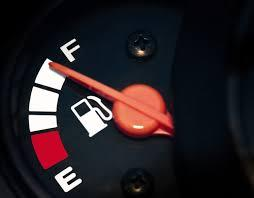We would be pleased if you find an error in the word problem, spelling mistakes, or inaccuracies and send it to us. Thank you!Tips to related online calculators
Do you have a system of equations and looking for calculator system of linear equations?
Tip: Our volume units converter will help you with the conversion of volume units.

## Next similar math problems:

• Gasoline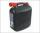35 l of gasoline is to be divided into four canisters so that in the third canister was 5 l less than the first canister, the fourth canister 10 l more than in the third canister and the second canister half what in the first canister. How many liters of
• PoolIf water flows into the pool by two inlets, fill the whole for 19 hours. The first inlet filled pool 5 hour longer than the second. How long pool take to fill with two inlets separately?
• Three workers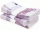The three workers received € 2,850 together for the work done. They divided them according to the time worked so that the first received 20% less than the second and the third € 50 more than the second. How much EUR did each worker receive?
• 925 USDFour classmates saved an annual total 925 USD. The second save twice as the first, third 35 USD more than the second and fourth 10 USD less than the first. How USD save each of them?
• Three friends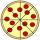Divide 570 euros to three friends so that first will get 50 euros less than the second and third twice more than the first. How many euros will get everyone?
• RemunerationTotal remuneration for workers is 47 000 CZK. The second worker received 20% more than the first and third 4000 CZK less than a second employee. How many CZK each received?
• Potatoes bags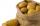I have three bags with 21 kg potatoes. The first bag is 5.5 kg more than in the second bag and the third is 0.5 kg more than in the second bag. Determine how many kgs of potatoes is in each bag.
• RewardThree workers have shared a common reward 13110 CZK follows: first worker got 35% less than the second and third worker got 20% more than the second worker. How much got each worker?
• Warehouses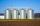In the three warehouses, a total of 70 tons of grain was stored. In the second warehouse was stored 8.5t less and in the third 3.5t more than in the first. How many tons of grain was stored in each warehouse?
• MilkThere were 22 liters of milk in three containers. There was 6 liters more in the first container than in the second. After pouring 5 liters from the first container into the third container, the same quantity of milk is in the second and third container.
• TrioTrio of workers earn 750 euros. Money divided by the work that each of them made​​. First received twice as the second, the second received three times more than the third. How many got everyone from workers?
• RepairingThree employees earned a total of € 469 for repairing the equipment. They split so that the first got 20% more than the second, and the third 15% more than the second. How many euros did everyone get?
• ContestantsThe three best contestants are to divide the total prize of CZK 4,200. The second gets 20% more than the third. And the first one gets 200 CZK less than the second and the third together. How much will everyone get?
• Part-time workersThree part-time workers received CZK 1,235 for their work. The first received 20% less than the second and the third received 45 CZK more than the second. Determine how many crowns (CZK) each of them received.
• Crowns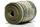1180 crowns is divided into three people that A got 20% less than B and C by 15% more than B. How many got A,B and C?
• Scouts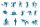Three scouts went on a three day trip. The second day went 4 km more than the first day. The third day went two times less than the first day. They went along 54 km. How many kilometers went every day together?
• Boxes200 boxes have been straightened in three rows. The first was 13 more than in the second, and in the second was one fifth more than in the third one. How many boxes are in each row?